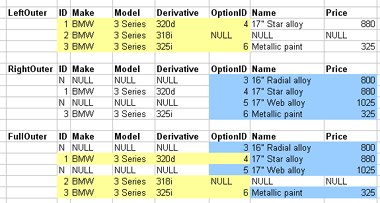# 1.3. Joins

In this lecture we look at...
[Section notes PDF 233Kb].

# 1.3.01. Introduction

• Recap: pulling data out of individual relations
• By row, by column
• Select and project
• Access across multiple relations
• Miniworld approximation
• Fragmenting entities by cardinality
• Tuples as entity fragments
• Relationships within relations
• Joins
• Join types (condition and unmatched)

# 1.3.02. Access across relations

• Relational model allows multiple relations to exist within one database schema
• Relations can be accessed individually or together (joins).
• Referential integrity
• Relations relating
• Pulling data out of single relations
• Select and project
• Pulling related data out of
• Multiple relations using Join

# 1.3.03. Miniworld approximation

• Universe of Discourse, or Miniworld
• Miniworld is an incomplete model of the real world
• The relational data model as a model for the miniworld
• Approximation
• Separate and distinct entities
• Single complex entities
• Separate related entities
• Cardinality of relationships
• Each relation made up of attributes
• Values can be used as references

# 1.3.04. Pointing mechanism

• Relation has a Primary key
• Tuple contains Primary key value
• Foreign keys
• Tuples can contain a reference to another relation's Primary key
• Just numbers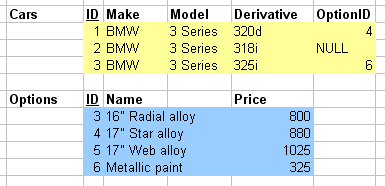One number identifies a single tuple in one relation (local), one number identifies a single tuple in another relation (foreign).

# 1.3.04b. Pointing mechanism example in C

• C programming language
```int a=0;
int b=0;
a = &b;
```
• a points to b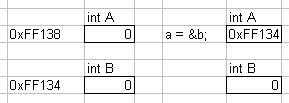In databases, typically done with unique identifiers (IDs) rather than memory addresses.

# 1.3.04c. Pointing mechanism with structures

• Foreign key importing
```typedef struct car
{
int ID;
char[] make;
char[] model;
char[] derivative;
int optionID;
} car;

typedef struct option
{
int ID;
char[] name;
int price;
} option;

car c;
option o;
//...data structure populating
c.optionID = o.ID;
```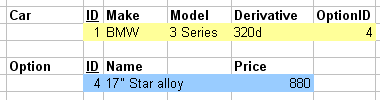# 1.3.05. Relational cardinality

• 1:0 relationships
• Single entity
• Uniquely indentifiable
• Candidate keys
• Primary Key
• 1:1 relationships
• Two entities, A and B
• 1 A relates to 1 B and vice versa
• 1:N relationships
• M:N relationships

# 1.3.06. Relationships in the relational model

• Two relations, A and B
• A side, B side, 1 side, N side
• 1:1 relationships
• Key can go on either side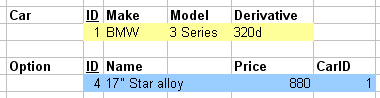• 1:N relationships
• Key cannot go on 1 side
• Has to go on N side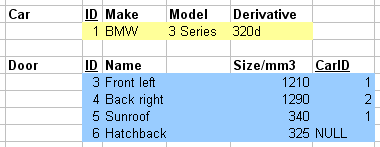• M:N relationships
• Nowhere obvious for the key to go
• Create new pairing relation

# 1.3.07. Joins

• Phase change, different point in lifecycle
• Join operation
• Combines related tuples, conditionally
• From two relations
• Into single tuples
• Allows processing of relationships
• Among multiple relations

# 1.3.08. Joins, canonical algebraic form

• Conditional (on join condition)
• Only combines tuples where true
• Cartesian product (conditionless)
• example of conditionless join
• all tuples combined
• R ⋈true S
• ⋈, Binary operator
e.g. R ⋈<join_condition> S

# 1.3.09. Join equivalence

• Equivalent to sequence
• Cartesian product (X)
• followed by Selection (s)
• ACTUAL_DEPENDENTS =
sSSN=ESSN(EMPNAMES X DEPENDENT)
• or
• ACTUAL_DEPENDENTS =
EMPNAMES ⋈ SSN=ESSN(DEPENDENT)

# 1.3.10. Join types (condition)

• Theta: Ai q Bj
(A from R, B from S)
• q is comparison operator
=,<,>,!=,>=
• Ai and Bj share the same domain
• Equi: Ai = Bj
• Theta join where q is =
• Natural: Ai and Bj are the same attribute
• in two separate relations (name and domain)
• * denotes natural join
• e.g. EMPNAMES * DEPENDENTS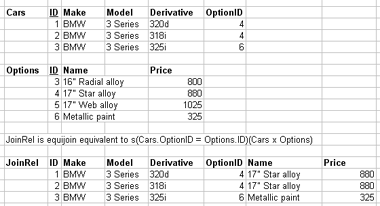# 1.3.11. Join types (inner and outer)

• Inner joins
• not the only joins
• eliminate tuples without a matching counterpart
• i.e. tuples with a null value for the join attribute are discarded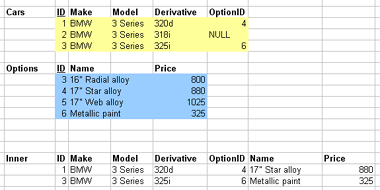# 1.3.12. Outer joins

• Outer joins control what's discarded
• Keep unmatched tuples in either
• Left, right, or both relations
• Left, right of full outer join correspondingly